Ex 5.3

Chapter 5 Class 6 Understanding Elementary Shapes
Serial order wise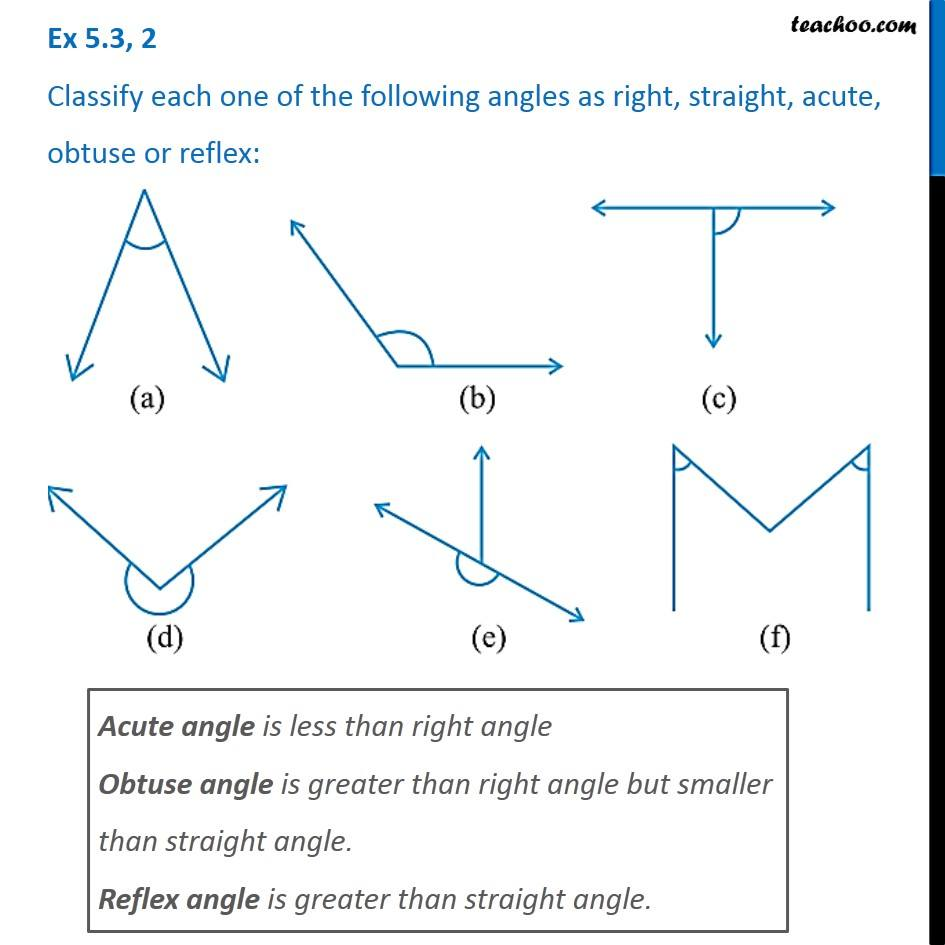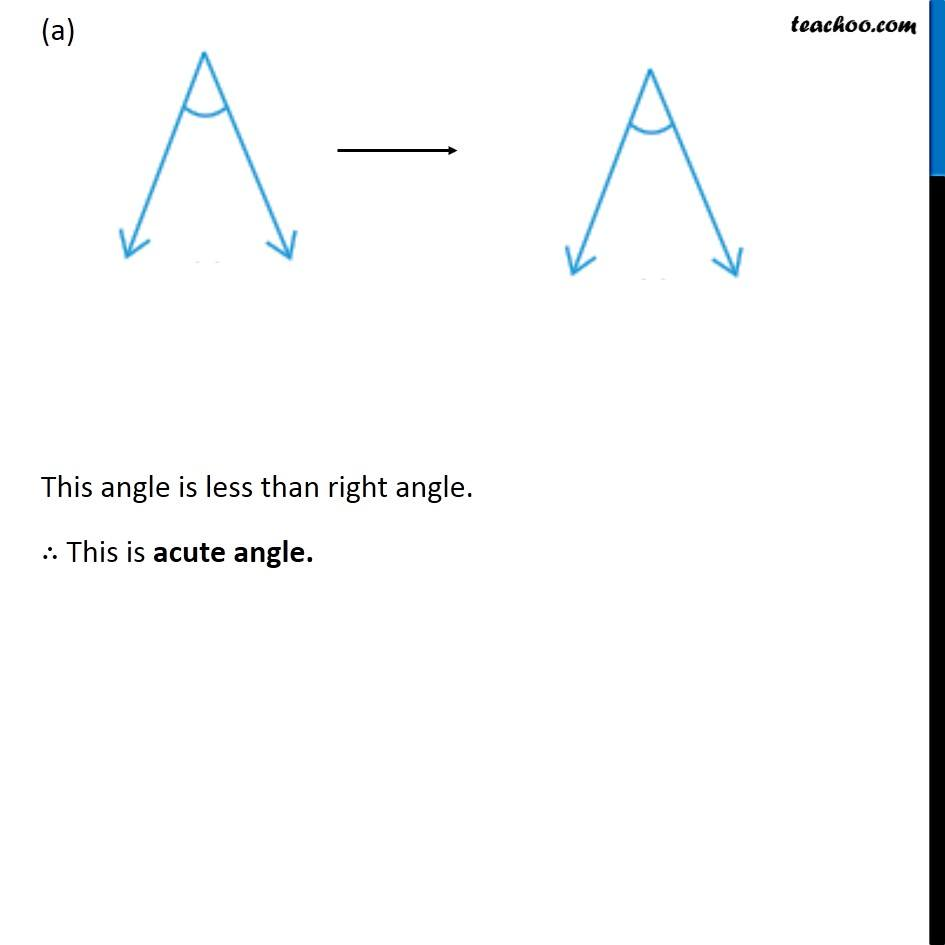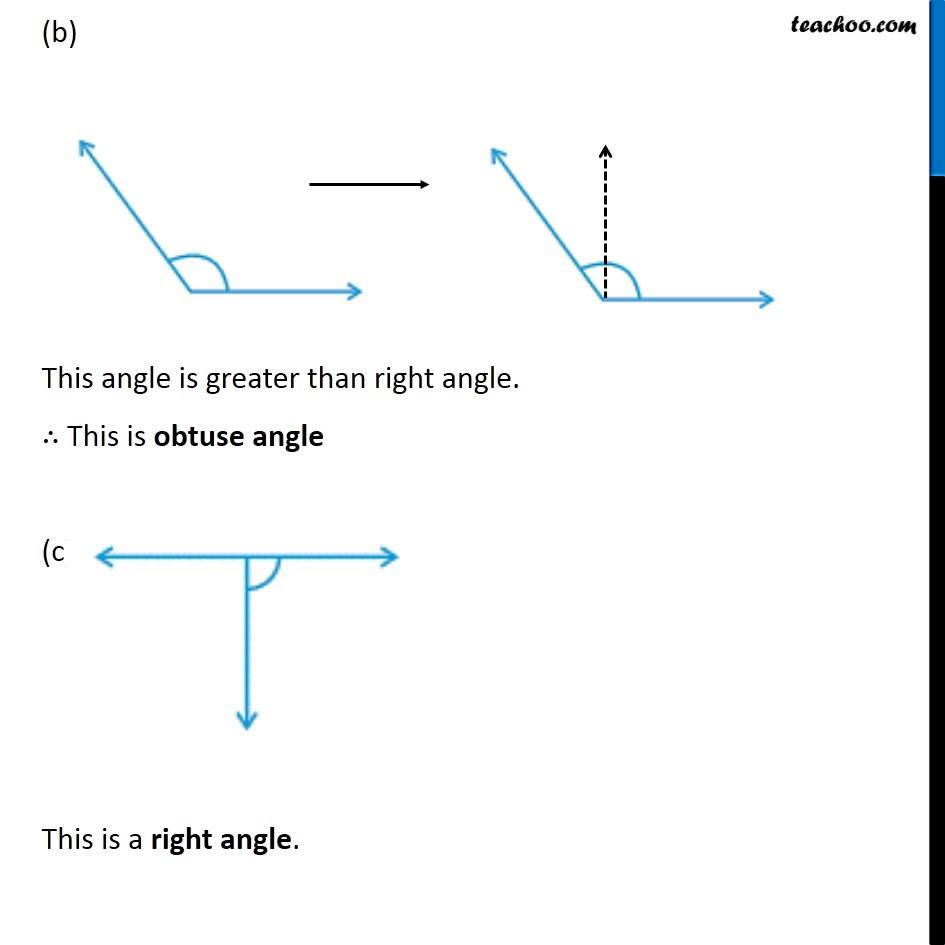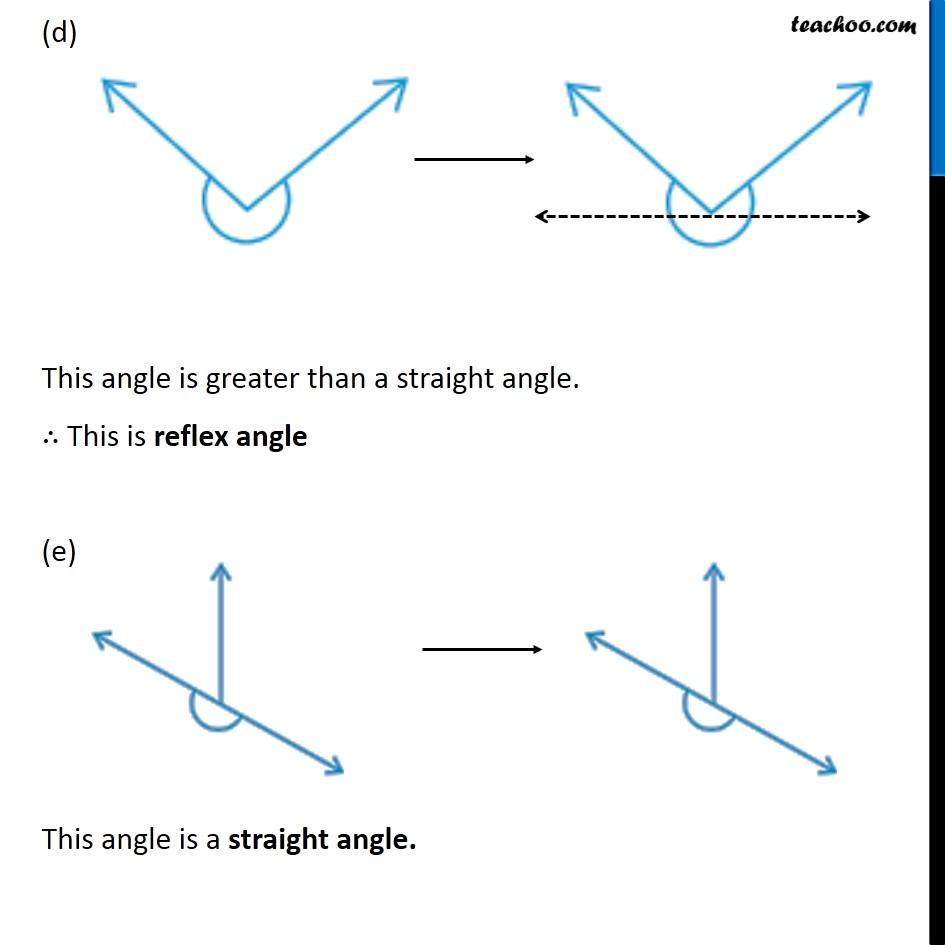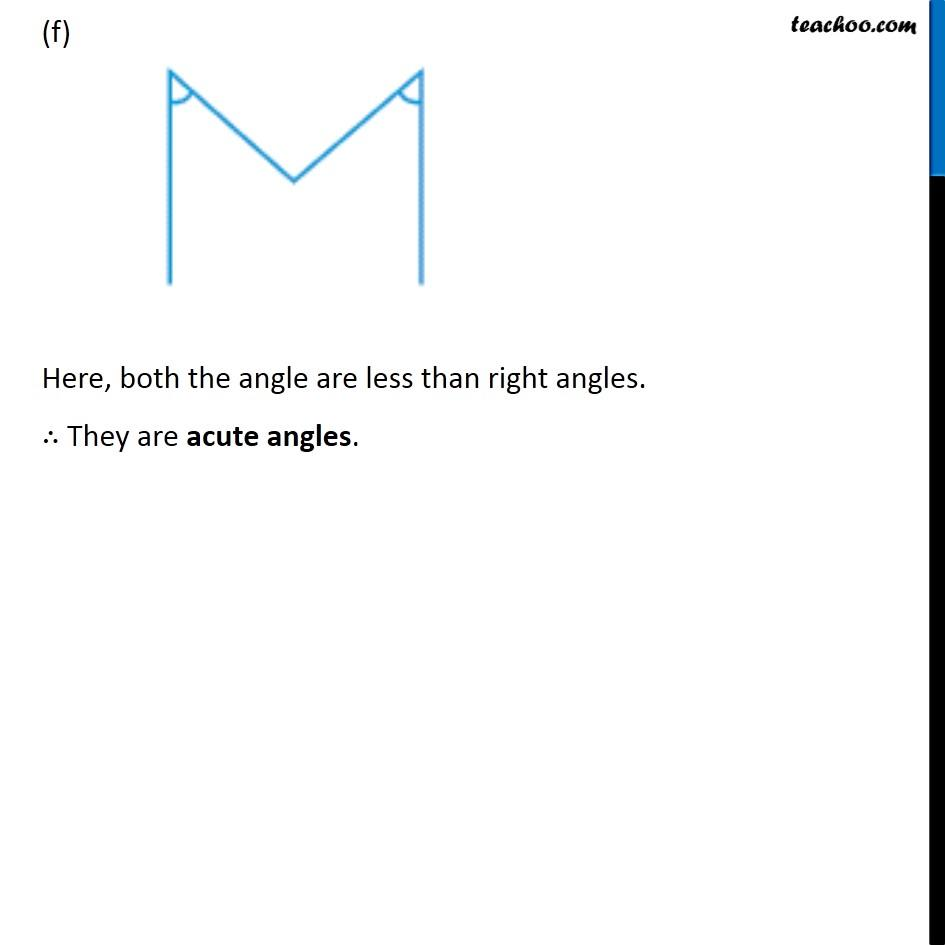Learn in your speed, with individual attention - Teachoo Maths 1-on-1 Class

### Transcript

Ex 5.3, 2 Classify each one of the following angles as right, straight, acute, obtuse or reflex: Acute angle is less than right angle Obtuse angle is greater than right angle but smaller than straight angle. Reflex angle is greater than straight angle. (a) This angle is less than right angle. ∴ This is acute angle. (b) This angle is greater than right angle. ∴ This is obtuse angle (c) This is a right angle. (d) This angle is greater than a straight angle. ∴ This is reflex angle (e) This angle is a straight angle. (f) Here, both the angle are less than right angles. ∴ They are acute angles.